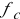# Day 167

• Radiation emitted by any object, dependent on an object’s properties
• Black body is an object that absorbs all incident light upon it
• Radiated energy varies with λ and T.
• As T increases, total energy increases
• As T increases there is a shift to shorter λs

### Wien’s Displacement Law

• λmax T = 0.2898 x 10-2 mK
• This led to disagreement between classical and quantum mechanics at short wavelengths
• Experimental data shows that as λ approaches 0, energy approaches 0
• In theory, as λ approaches 0, energy should approach infinity
• This contradiction is called the “ultraviolet catastrophe”

### Plank’s Solution

• Max Planck solved the dilemma with the theory that blackbody radiation was produced by “resonators” which are submicroscopic electric oscillators following the equation
• En=nhf where n = quantum number, f = frequency of vibration of the resonators and h = his constant 6.626 x 10-34 Js

• Resonators emit energy in discrete bundles of energy called “quanta” or “photons” by jumping from one energy state to another
• Energy of a light quantum is the energy difference between two adjacent energy levels
• E = hf determines the energy of a photon of a certain frequency

## The Photoelectric Effect

### Definition of Photoelectric Effect

• The effect of emitting electrons from the surface of a metal due to incident light
• The emitted electrons are called photoelectrons
• First discovered by Hertz
• Later explained by Einstein in 1905

### Einstein’s Explanation – Point One

• No electrons will be emitted when light falls below the cutoff frequency, f0 (dependent on material)
• This is not dependent on intensity
• This explanation contradicts the wave theory which was prevalent at the time of the photoelectric effect discovery

### Einstein’s Explanation – Point Two

• If the frequency of the incident light is greater than the cutoff frequency (f>f0), PE is observed and the number of photoelectrons is proportional to the intensity of the light
• The maximum kinetic energy is independent of intensity (doesn’t fit the classical model)

### Einstein’s Explanation – Points Three and Four

• Maximum kinetic energy increases with frequency
• Photoelectric effect occurs almost instantaneously (doesn’t fit classical model either)

### Einstein Extends Plank’s Hypothesis

• Einstein extended Planck’s theory to all electromagnetic waves because they can be considered to be a stream of photons

### The “Work Function”

• Einstein theorized that electrons must overcome a barrier when escaping from the surface of the metal and would need energy to do so
• He called this the “work function” (Φ)

### The Photoelectric Equation

• KEmax = hf – Φ
• Where KE = kinetic energy in J
• h = Planck’s constant (6.63 x 10-34 Js)
• Or h = 4.14 x 10-15 eVs
• f = frequency in Hz
• Φ = work function in eV or J

### Explanation of Equation

• PE not observed below certain frequency because energy of incoming photon must exceed Φ
• KEmax is independent of intensity because intensity is NOT part of PE equation
• KEmax increases with increased frequency because of equation
• Electrons are emitted almost instantaneously because of one-on-one interactions between particles

### Graph of KEmax vs. Frequency

• Shows a direct relationship between KE and frequency
• KEmax = 0 refers to the cutoff frequency, fc
• The slope of the line is “h”, Planck’s constant
•= Φ /h and λc = c/fc = c/(Φ/h) = (hc)/ Φ; wavelengths greater than λ do not yield the PE

(source)